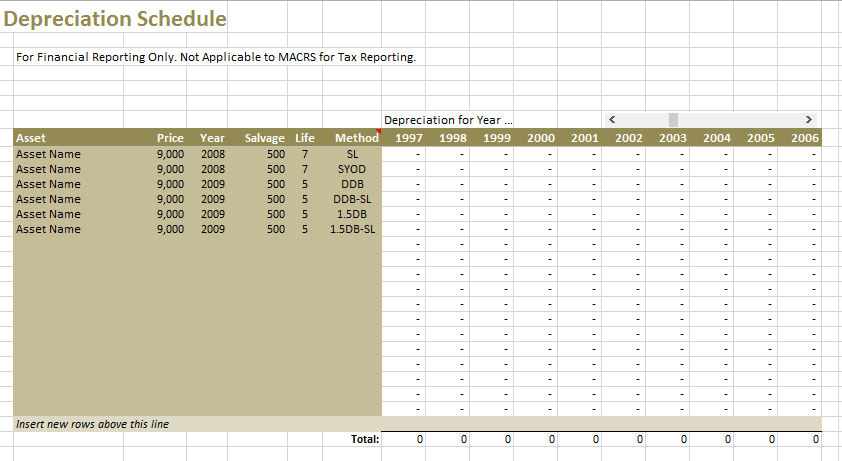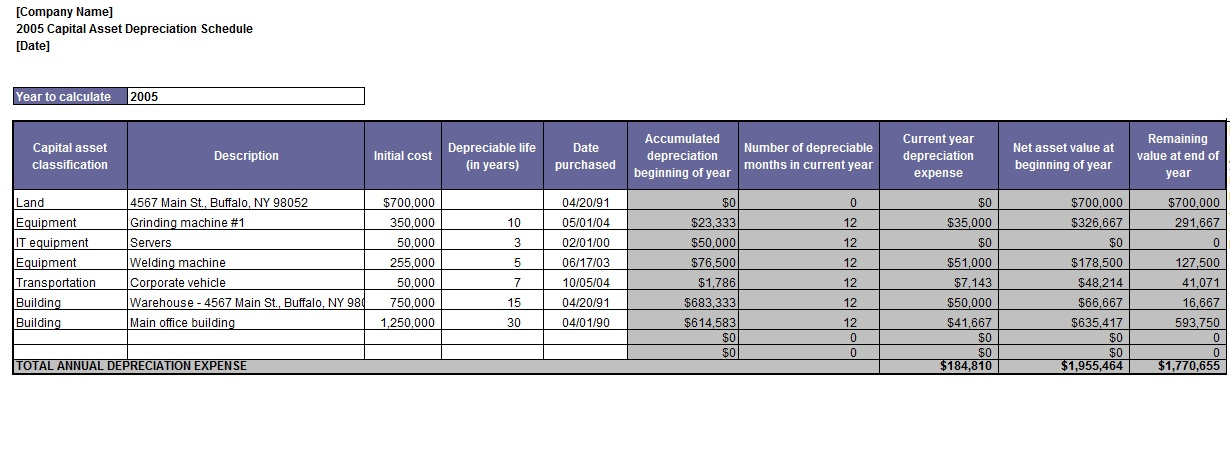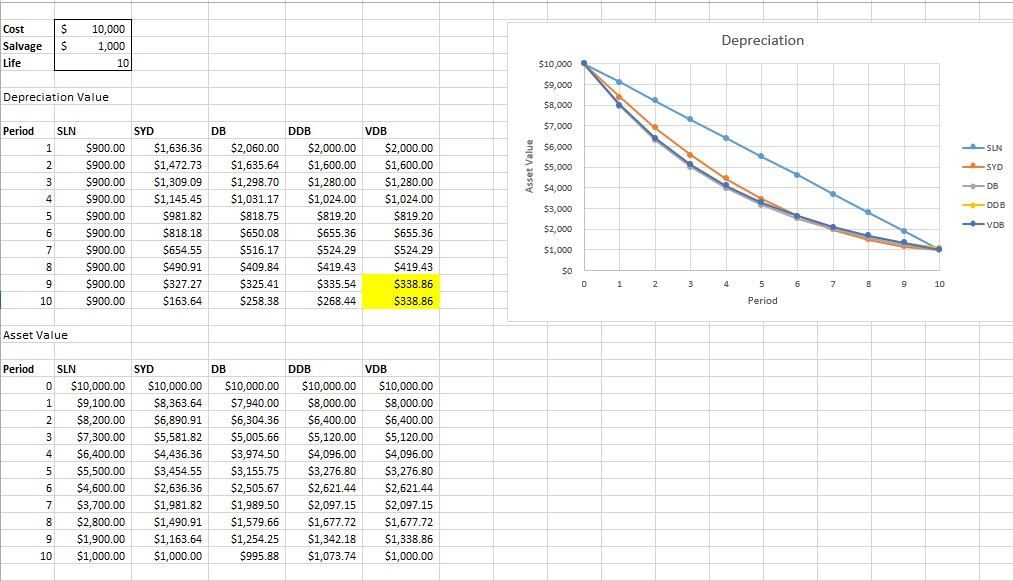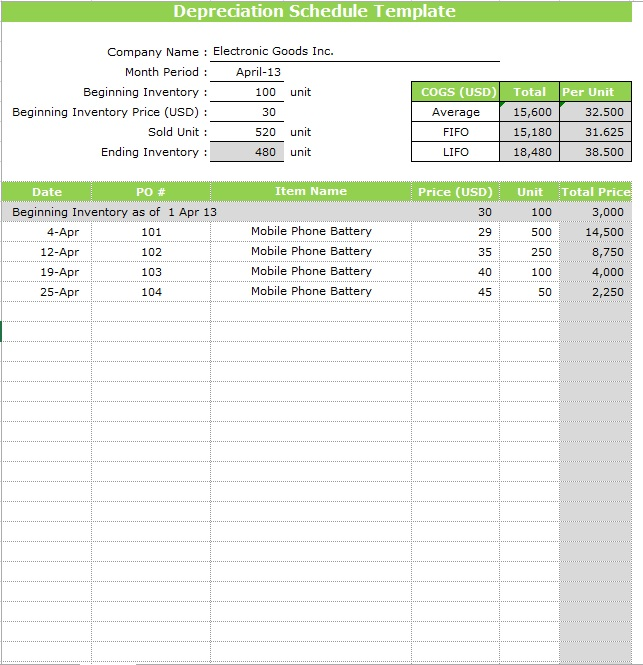# Depreciation Schedule Templates

• Home
• Depreciation Schedule Templates### Depreciation Schedule Templates

• July 25, 2017

Depreciation is basically a non-cash expense that is carried out as a loss in value of a particular asset because of usage. A Depreciation Schedule Template is used to create depreciation schedules that are widely used in the business world for tracking accounting records because a financial statement of a business is based on the total value of assets and total accumulated amount of depreciation. For creating and managing a depreciation schedule, usually there is a particular person who is responsible for all these things, whereas in large companies there is a separate group of people working as full time managers of depreciation schedules. A better way of creating and maintaining a depreciation schedule is to create separate schedules for each asset. For example, in a large business entity, the assets can be buildings, machinery, furniture, office equipment, hardware, software and much more.

# Free Depreciation Schedule Templates

Here is preview of this Depreciation Schedule Template,Depreciation schedules are mostly created by professional accountants using different methods. Following tips will help you in creating a depreciation schedule with ease.

• First of all choose an appropriate method of calculating depreciation. There are lots of different methods however, the three very common and widely used depreciation methods are the straight line methods, sum of year method and the declining balance method. All these methods are good and effective however the straight line method is the simplest and easiest method.
• List all the different types of assets for which you want to create a depreciation schedule. It is good and convenient to create separate schedules for each asset. You can create separate schedules in one Excel book using separate sheets or can even create separate files.
• Open a spreadsheet program and create a depreciation table by writing different headings in the top row including the year (end of), the value of assets, Depreciation expenses and cumulative Depreciation.
• Type all the relative information under each category. Under the heading of the year, write writing years from the current year to the year you want to make depreciation. For example, if the duration of the depreciation is 3 years and the current year is 2005, the column will include years 2005, 2006, 2007 and 2008.
• In the value of asset column, type the full value of the asset. In the remaining two rows type “0”.
• In the next year row for the first year, in the column of “depreciation expanse”, write the total amount that you want to depreciate. First calculate the amount using your preferred method. Subtract that particular value from the total amount of asset and write the difference in the column of “value of asset”.
• Similarly fill all the columns using appropriate numbers that you get from the calculations.

Additional to all these things, also include the information that is important for the determination of annual depreciation including original purchase date, recovery period and cost basis etc. The purpose of using depreciation schedule is more than tracking your accounting books. These schedules will help you in assessing the age of your assets and will tell you what will be the perfect time to replace your asset without getting any kind of loss.Here is preview of this Depreciation Schedule Template,Here is preview of this Depreciation Schedule Template,Here is preview of this Depreciation Schedule Template,Here is preview of this Depreciation Schedule Template,Here is preview of this Depreciation Schedule Template,Here is preview of this Depreciation Schedule Template,Here is preview of this Depreciation Schedule Template,